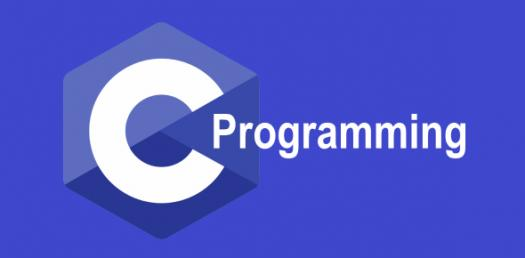# C Programming Coding Questions! Trivia Quiz

10 Questions | Total Attempts: 705SettingsThe trivia quiz below is made up of c programming coding questions! Programming in C is fairly easy because it uses basic commands in English; this language is one of the most commonly used in a multitude of applications, including advanced scientific systems and operating systems. Refresh your understanding of this programming language by taking this short quiz. Good luck!

• 1.
Print the output of this program  void main() {        int  a, b, c, abc = 0;          a = b = c = 40;          if (c) {                int abc;                abc = a*b+c;         }         printf ("c = %d, abc = %d ", c, abc); }
• A.

C = 0 and abc = 40

• B.

C = 0 and abc = 0

• C.

C = 40 and abc = 0

• D.

NONE OF THESE

• 2.
What is wrong with this macro to find out the square of a number #define square(a) a*a?
• 3.
What will be output of the following program ?  void main() {          int cnt = 5, a;          do {                a /= cnt;         } while (cnt --);         printf ("%d ", a); }
• A.

RUNTIME ERROR

• B.

SYNTAX ERROR

• C.

COMPILATION ERROR

• D.

NO ERROR

• 4.
What will be the output of the following Printf function?         printf("%d",printf("%d",printf("%d",printf("%s","ILOVECPROGRAM"))));
• 5.
Deallocate memory without using free() in C.
• 6.
The following is a piece of C code, whose intention was to print a minus sign 20 times. But you can notice that, it doesn't work. #include int main() { int i; int n = 20; for( i = 0; i < n; i-- ) printf("-"); return 0; } Well fixing the above code is straight-forward. To make the problem interesting, you have to fix the above code, by changing exactly one character. There are 2 known solutions. See if you can get all those 2. one solution is to use n-- instead of i--.
• 7.
Write a “Hello World” program in ‘C’ without using a semicolon.
• 8.
Will the following program compile successfully? int main() { int a=10; int &k; k=a; }
• 9.
What will be the output of the program below #include void fun(int *a, int *b) { int *t; t=a; a=b; b=t; } main() { int a=2; int b=3; fun(&a,&b); printf(%d, %d",a, b); } a) 3, 2 b) compilation error c) error at run time d) 2, 3 e) None of the above
• A.

A

• B.

B

• C.

C

• D.

D

• E.

E

• 10.
Predict the ouput: #include void f() {  a=10;  printf("%d",a); } int a=5; main() {  a++,b++;  printf("%d,%d",a,b);  f(); }
Related TopicsBack to top